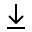MathBin Number Member

A number is a mathematical object used to count, measure and label. The original examples are the natural numbers 1, 2, 3, and so forth. A notational symbol that represents a number is called a numeral. The notion of number has been extended over the centuries to include 0, negative numbers, rational numbers such as $\frac{1}{2}$ and $-\frac{2}{3}$, real numbers such as $\sqrt{2}$ and pi (π), complex numbers, which extend the real numbers by including ⅈ, and sometimes additional objects. Calculations with numbers are done with arithmetical operations, the most familiar being addition, subtraction, multiplication, division, and exponentiation. Their study or usage is called arithmetic. The same term may also refer to number theory, the study of the properties of the natural numbers.

ℕ Counting numbers which may optionally start at zero, then 1, 2, 3, and so on. Natural numbers, their negative counterparts, and zero if you didn't already "count" it. Numbers that can be expressed as a fraction with an integer numerator and a positive integer denominator of the form m / n. All the measuring numbers, along with irrational numbers. Irrational numbers cannot be expressed as a fraction, such as pi (π) and the square root of two ($\sqrt{2}$). All real numbers, along with numbers that have an imaginary component. Complex numbers have the form a + b ⅈ, where ⅈ is the square root of negative one.

Topics